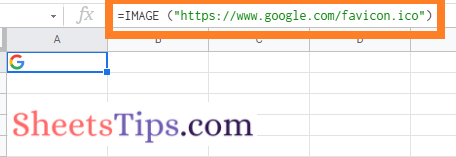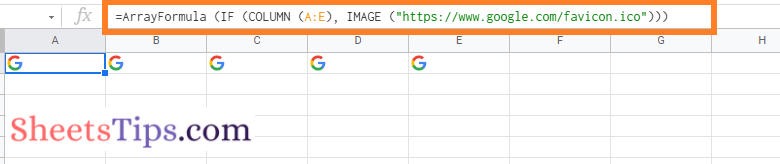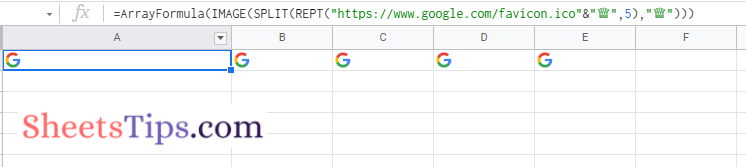# How to Repeat Images With an Array Formula in Google Sheets? Repeating Images with a Single Formula

With the help of Google Sheets, one of the great collaboration tools, we can perform marvelous operations. The spreadsheet software developed by Google comes in really handy when we want to perform complicated operations. For example, let’s assume that we want to insert an image into Google Sheets, which should appear across the cells only a specified number of times. To execute this, we need to use only single-cell formulas. So, in this post, let us learn how to create a repeating pattern in a spreadsheet using Google Sheets Tips and Tricks. Read further to find out more.

## Repeating Images with Array Formula

Let us consider that we have the image URL of the Google Logo. Now we have inserted the image by using the URL in Cell A1 as shown below. Now our challenge is to repeat this image the number of times specified with a single array formula. Let us learn how to get this done in the Google Spreadsheet in the next section.

### How to Create an Array Formula for Images in a Spreadsheet?

• 2nd Step: Now move to the cell and insert the image using the formula “=IMAGE (“https://www.google.com/favicon.ico”)“. In this example, I am using cell A1.• 3rd Step: Next, we need the same image to repeat the number of times we specify. In this post, let’s say I need to repeat the image five times. To move the cell, double click to modify the existing formula.
• 4th Step: Replace the existing formula with “=ArrayFormula (IF (COLUMN (A: E), IMAGE (“https://www.google.com/favicon.ico”))“.
• 5th Step: Press the “Enter” button and you will see the results as shown in the image below.That’s it. We got the desired results.

When you use ArrayFormula with COLUMN (A: E), you’ll get an array of numbers from 1 to 5: 1,2,3,4,5. The IF statement interprets the integers as TRUE values, so the picture is printed 5 times. For clarity, we may eliminate the FALSE value of the IF statement for clarity’s sake. We don’t use it.

### Method 2: Repeating Images Using the REPT Function in Google Sheets

Let us use the REPT function in the following method to repeat the images on Google Sheets. The steps to repeat the images on Google Sheets using the REPT function are as follows: